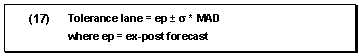# Forecast Formulas

Forecast Formulas source help.sap.com

The statistical forecast is based on several types of formula:

• Formulas on which the forecast models are based
• Formulas that are used to evaluate the forecast results
• Formula to calculate the tolerance lane for automatic outlier correction

Formulas for Forecast Models

Moving Average Model

This model is used to exclude irregularities in the time series pattern. The average of the n last time series values is calculated. The average can always be calculated from n values according to formula (1).

Formula for the Moving Average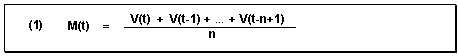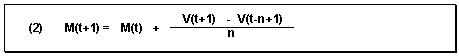Thus, the new average is calculated from the previous average value and the current value weighted with 1/n, minus the oldest value weighted with 1/n.

This procedure is only suitable for time series that are constant, that is, for time series with no trend-like or season-like patterns. As all historical data is equally weighted with the factor 1/n, it takes precisely n periods for the forecast to adapt to a possible level change.

Weighted Moving Average Model

You achieve better results than those obtained with the moving average model by introducing weighting factors for each historical value. In the weighted moving average model, every historical value is weighted with the factor R. The sum of the weighting factors is 1 (see formulas (3) and (4) below).

Formula for the Weighted Moving Average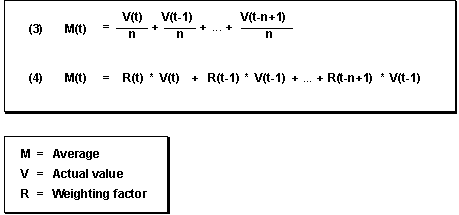If the time series to be forecasted contains trend-like variations, you will achieve better results by using the weighted moving average model rather than the moving average model. The weighted moving average model weighs recent data more heavily than older data when determining the average, provided you have selected the weighting factors accordingly. Therefore, the system is able to react more quickly to a change in level.

The accuracy of this model depends largely on your choice of weighting factors. If the time series pattern changes, you must also adapt the weighting factors.

First-Order Exponential Smoothing Model

The principles behind this model are:

• The older the time series values, the less important they become for the calculation of the forecast.
• The present forecast error is taken into account in subsequent forecasts.

Constant Model

The exponential smoothing constant model can be derived from the above two considerations (see formula (5) below). In this case, the formula is used to calculate the basic value. A simple transformation produces the basic formula for exponential smoothing (see formula (6) below).

Formulas for Exponential Smoothing

Determining the Basic Value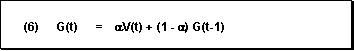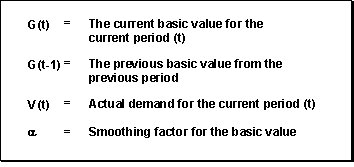To determine the forecast value, all you need is the preceding forecast value, the last historical value, and the “alpha” smoothing factor. This smoothing factor weights the more recent historical values more than the less recent ones, so they have a greater influence on the forecast.

How quickly the forecast reacts to a change in pattern depends on the smoothing factor. If you choose 0 for alpha, the new average will be equal to the old one. In this case, the basic value calculated previously remains; that is, the forecast does not react to current data. If you choose 1 for the alpha value, the new average will equal the last value in the time series.

The most common values for alpha lie, therefore, between 0.1 and 0.5. For example, an alpha value of 0.5 weights historical values as follows:

1st historical value: 50%

2nd historical value: 25%

3rd historical value: 12.5%

4th historical value: 6.25%

The weightings of historical data can be changed by a single parameter. Therefore, it is relatively easy to respond to changes in the time series.

The constant model of first-order exponential smoothing derived above can be applied to time series that do not have trend-like patterns or seasonal variations.

General Formula for First-Order Exponential Smoothing

Using the basic formula derived above (6), the general formula for first-order exponential smoothing (7) is determined by taking both trend and seasonal variations into account. Here, the basic value, the trend value, and the seasonal index are calculated as shown in formulas (8) – (10).

Formulas for First-Order Exponential Smoothing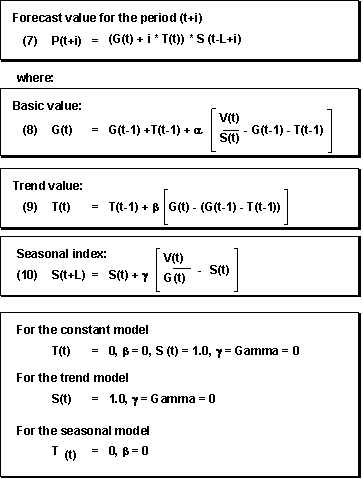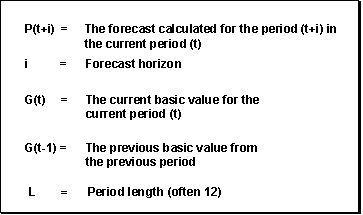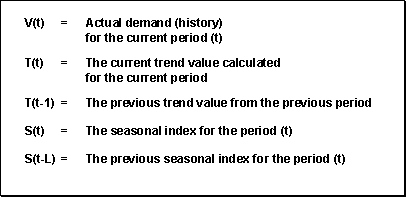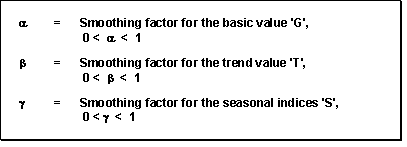Second-Order Exponential Smoothing Model

If, over several periods, a time series shows a change in the average value which corresponds to the trend model, the forecast values always lag behind the actual values by one or several periods in the first-order exponential smoothing procedure. You can achieve a more efficient adjustment of the forecast to the actual values pattern by using second-order exponential smoothing.

The second-order exponential smoothing model is based on a linear trend and consists of two equations (see formula (11)). The first equation corresponds to that of first-order exponential smoothing except for the bracketed indices. In the second equation, the values calculated in the first equation are used as initial values and are smoothed again.

Formulas for Second-Order Exponential Smoothing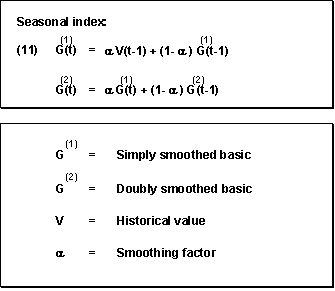Forecast Evaluation Criteria

Every forecast should provide some kind of basis for a decision. The SAP R/3 System calculates the following parameters for evaluating a forecast’s quality:

• Error total
• Tracking signal
• Theil coefficient

Error Total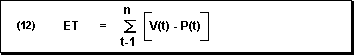Mean Absolute Deviation for Forecast Initialization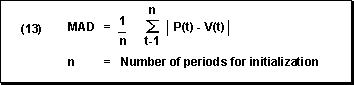Mean Absolute Deviation for Ex-Post Forecast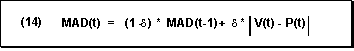Tracking Signal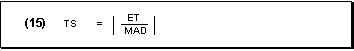Theil Coefficient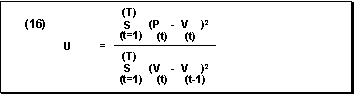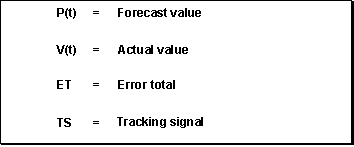Formula for the Tolerance Lane

To correct outliers automatically in the historical data on which the forecast is based, you select Outlier control in the forecast profile. The system then calculates a tolerance lane for the historical time series, based on the sigma factor. Historical data that lies outside the tolerance lane is corrected so that it corresponds to the ex-post value for that point in time. If you run the forecast online, historical data that has been automatically corrected by this function is indicated in column C of the Forecast: Historical Values dialog box.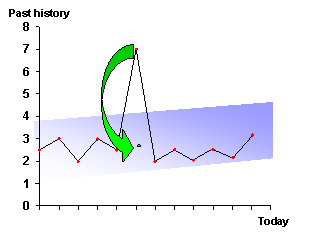The width of the tolerance lane for outlier control is defined by the sigma factor. The smaller the sigma factor, the greater the control. The default sigma factor is 1, which means that 90 % of the data remains uncorrected. If you set the sigma factor yourself, set it at between 0.6 and 2.

Tolerance Lane